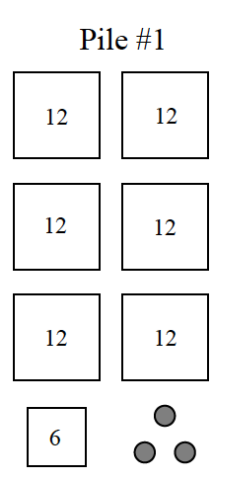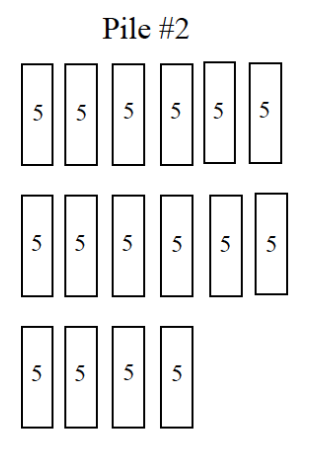### Home > ACC6 > Chapter 1 Unit 1 > Lesson CC1: 1.2.2 > Problem1-58

1-58.

The diagrams below represent piles of pennies.1. Which pile has more pennies? Explain your reasoning.

Try calculating the sum of each pile.

Don't forget to state which pile has more!

Pile #1 has $81$ pennies and Pile #2 has $80$ pennies.

2. Write two different numerical expressions to represent the number of pennies in each pile.

What different ways can you calculate the sums of the piles?

For instance: $\text{Pile #1} = (6)(12) + 6 + 3$

3. Write a number comparison statement(using $>$ , $<$ , or $=$ ) to show if the number of pennies in one pile is greater than the other or if they are the same.

Remember:
$>$ means that the number on the left is greater than the number on the right and $<$ means that the number on the left is less than the number on the right.